Скачать презентацию Structure and Synthesis of Robot Motion Making Sense

770db22c4cc277a2d5530223712cb60c.ppt

• Количество слайдов: 35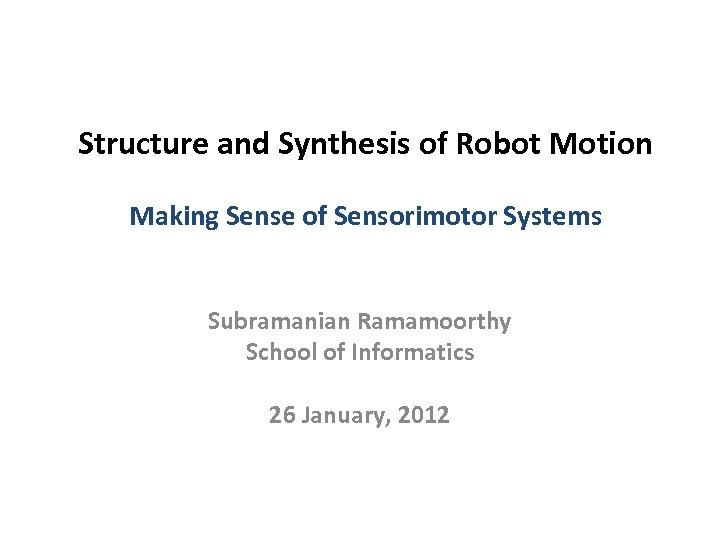Structure and Synthesis of Robot Motion Making Sense of Sensorimotor Systems Subramanian Ramamoorthy School of Informatics 26 January, 2012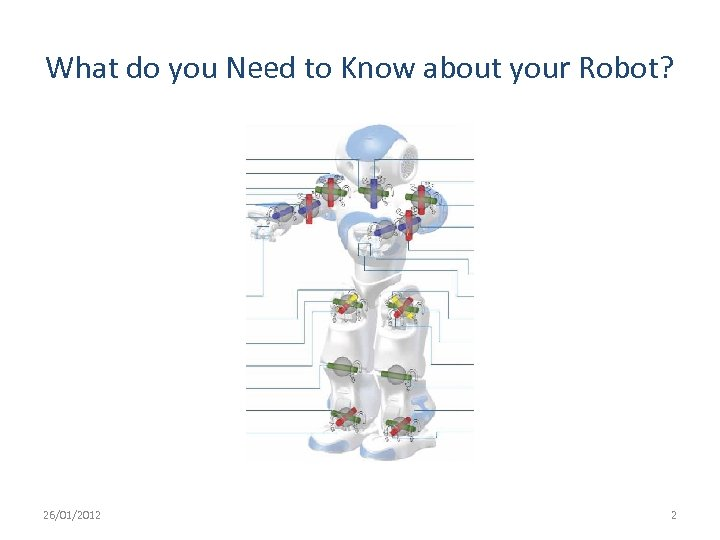What do you Need to Know about your Robot? 26/01/2012 2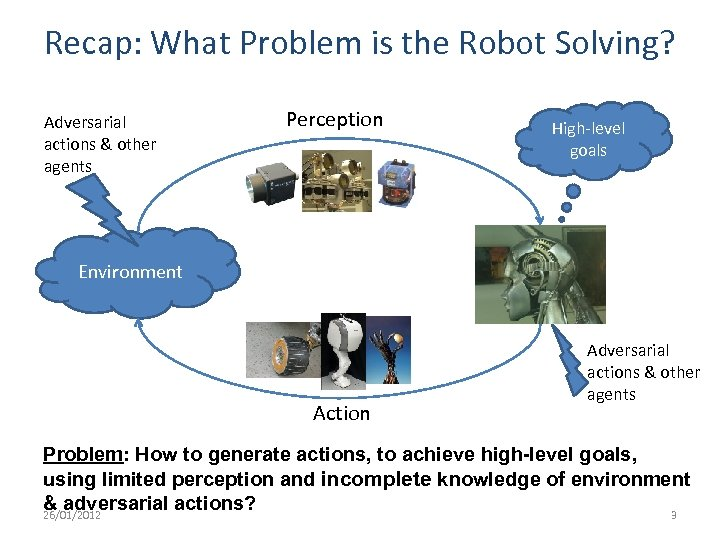Recap: What Problem is the Robot Solving? Adversarial actions & other agents Perception High-level goals Environment Action Adversarial actions & other agents Problem: How to generate actions, to achieve high-level goals, using limited perception and incomplete knowledge of environment & adversarial actions? 26/01/2012 3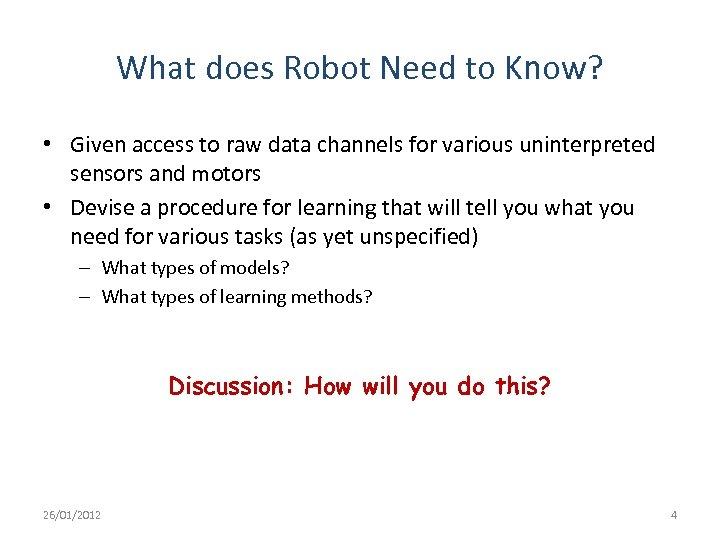What does Robot Need to Know? • Given access to raw data channels for various uninterpreted sensors and motors • Devise a procedure for learning that will tell you what you need for various tasks (as yet unspecified) – What types of models? – What types of learning methods? Discussion: How will you do this? 26/01/2012 4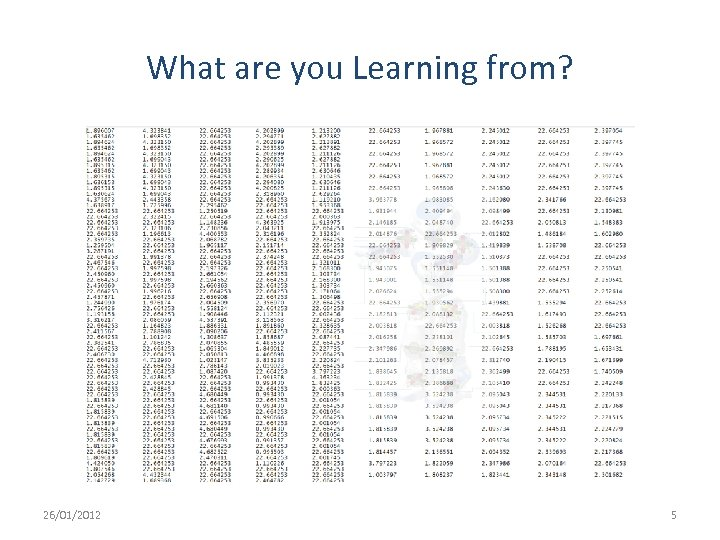What are you Learning from? 26/01/2012 5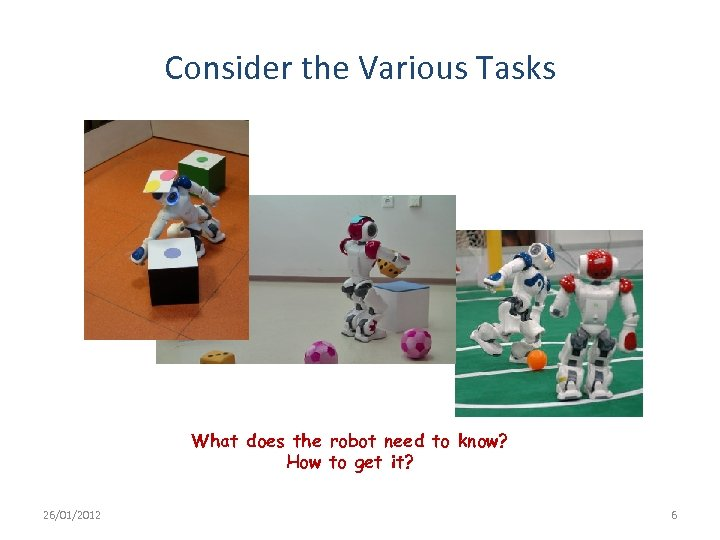Consider the Various Tasks What does the robot need to know? How to get it? 26/01/2012 6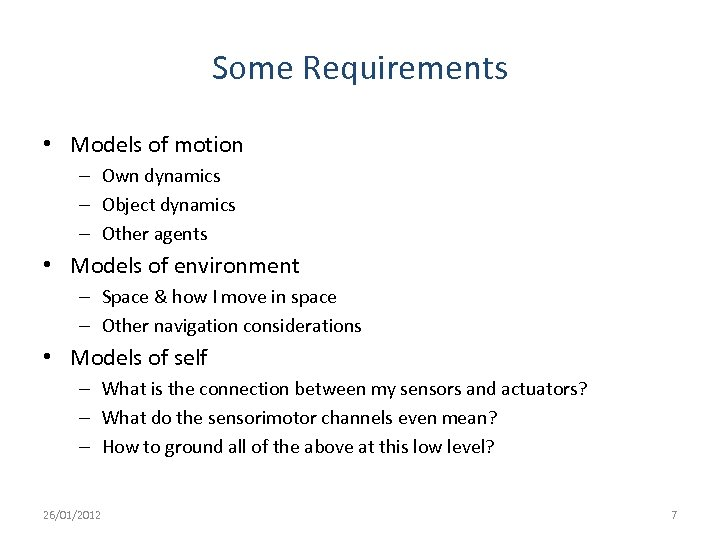Some Requirements • Models of motion – Own dynamics – Object dynamics – Other agents • Models of environment – Space & how I move in space – Other navigation considerations • Models of self – What is the connection between my sensors and actuators? – What do the sensorimotor channels even mean? – How to ground all of the above at this low level? 26/01/2012 7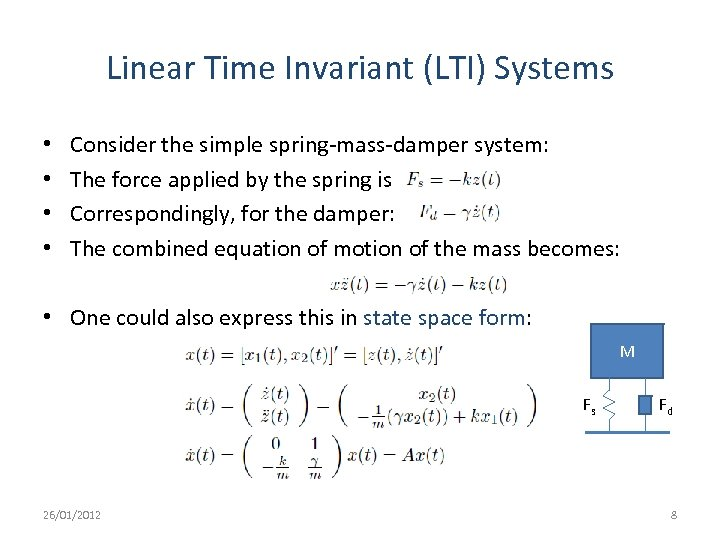Linear Time Invariant (LTI) Systems • • Consider the simple spring-mass-damper system: The force applied by the spring is Correspondingly, for the damper: The combined equation of motion of the mass becomes: • One could also express this in state space form: M Fs 26/01/2012 Fd 8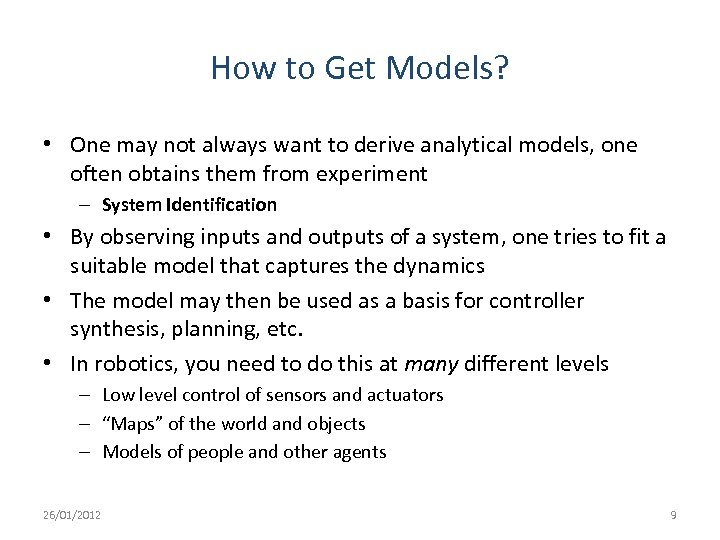How to Get Models? • One may not always want to derive analytical models, one often obtains them from experiment – System Identification • By observing inputs and outputs of a system, one tries to fit a suitable model that captures the dynamics • The model may then be used as a basis for controller synthesis, planning, etc. • In robotics, you need to do this at many different levels – Low level control of sensors and actuators – “Maps” of the world and objects – Models of people and other agents 26/01/2012 9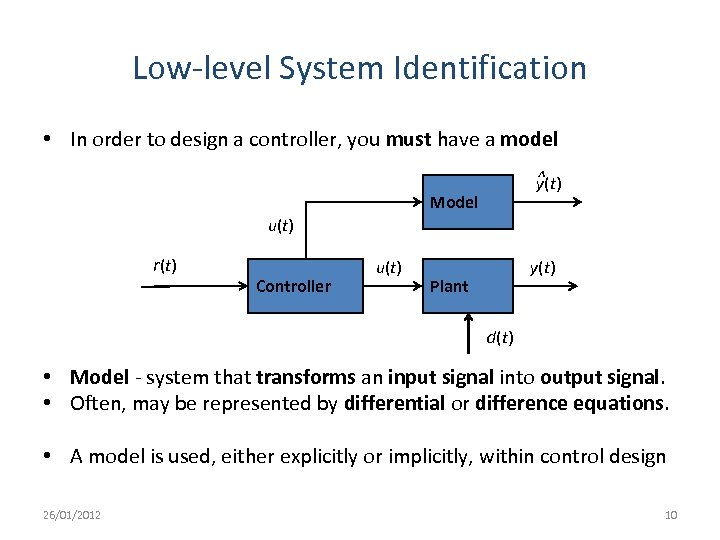Low-level System Identification • In order to design a controller, you must have a model ^ y(t) Model u(t) r(t) Controller u(t) y(t) Plant d(t) • Model - system that transforms an input signal into output signal. • Often, may be represented by differential or difference equations. • A model is used, either explicitly or implicitly, within control design 26/01/2012 10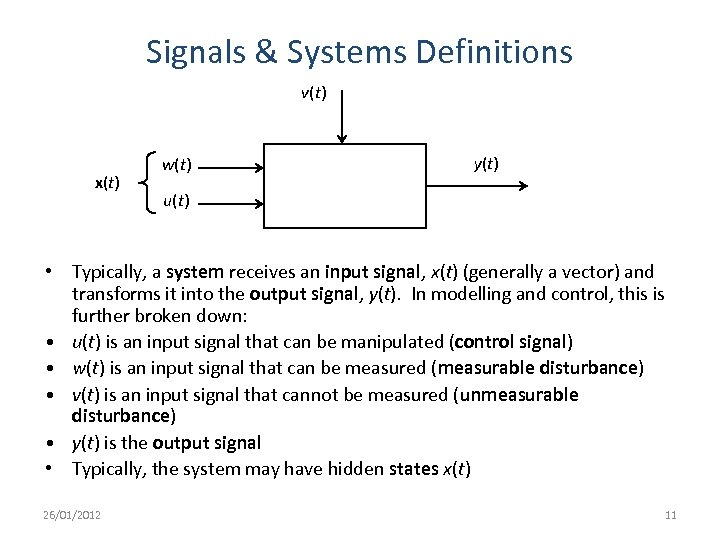Signals & Systems Definitions v(t) x(t) w(t) y(t) u(t) • Typically, a system receives an input signal, x(t) (generally a vector) and transforms it into the output signal, y(t). In modelling and control, this is further broken down: • u(t) is an input signal that can be manipulated (control signal) • w(t) is an input signal that can be measured (measurable disturbance) • v(t) is an input signal that cannot be measured (unmeasurable disturbance) • y(t) is the output signal • Typically, the system may have hidden states x(t) 26/01/2012 11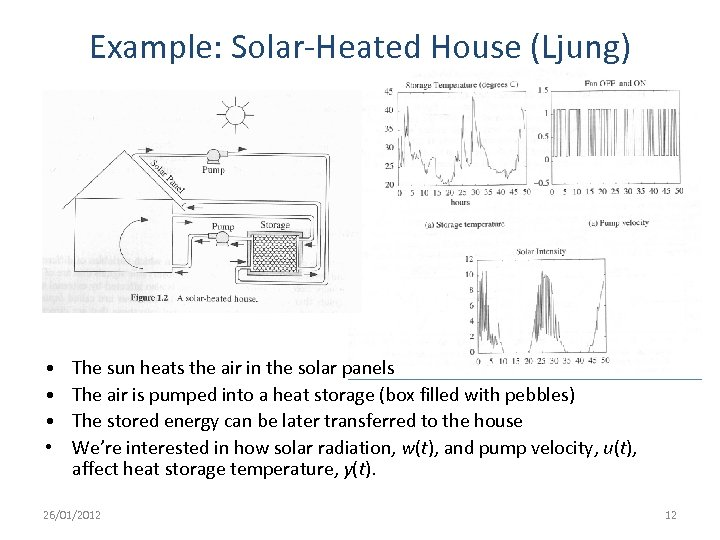Example: Solar-Heated House (Ljung) • • The sun heats the air in the solar panels The air is pumped into a heat storage (box filled with pebbles) The stored energy can be later transferred to the house We’re interested in how solar radiation, w(t), and pump velocity, u(t), affect heat storage temperature, y(t). 26/01/2012 12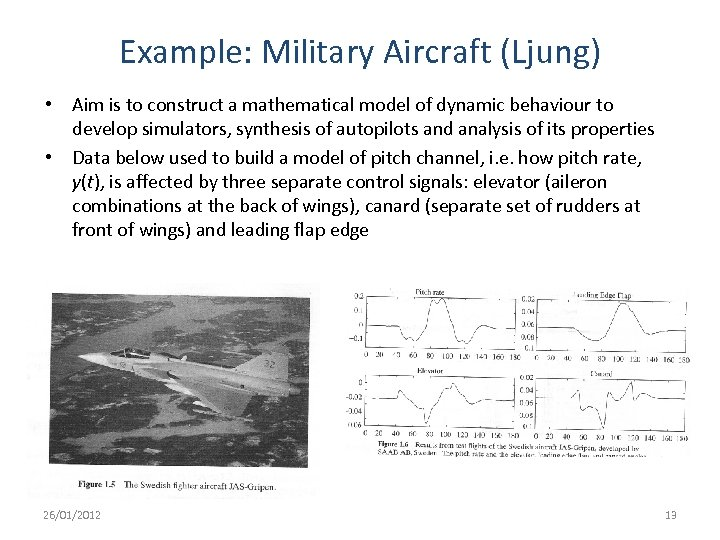Example: Military Aircraft (Ljung) • Aim is to construct a mathematical model of dynamic behaviour to develop simulators, synthesis of autopilots and analysis of its properties • Data below used to build a model of pitch channel, i. e. how pitch rate, y(t), is affected by three separate control signals: elevator (aileron combinations at the back of wings), canard (separate set of rudders at front of wings) and leading flap edge 26/01/2012 13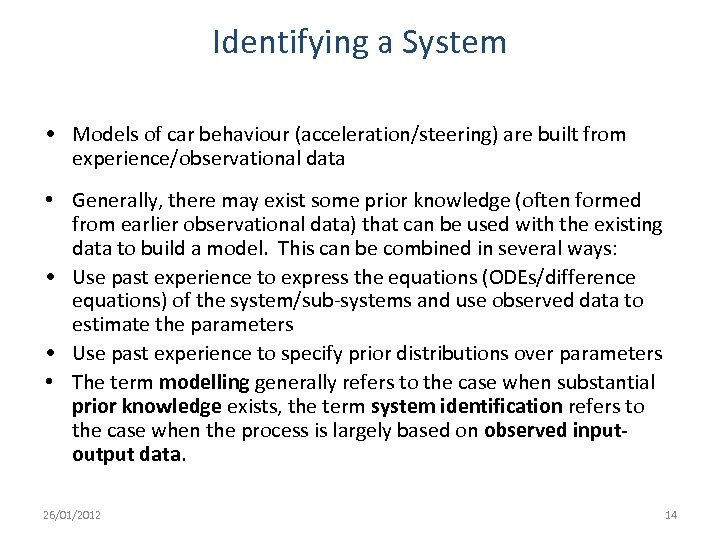Identifying a System • Models of car behaviour (acceleration/steering) are built from experience/observational data • Generally, there may exist some prior knowledge (often formed from earlier observational data) that can be used with the existing data to build a model. This can be combined in several ways: • Use past experience to express the equations (ODEs/difference equations) of the system/sub-systems and use observed data to estimate the parameters • Use past experience to specify prior distributions over parameters • The term modelling generally refers to the case when substantial prior knowledge exists, the term system identification refers to the case when the process is largely based on observed inputoutput data. 26/01/2012 14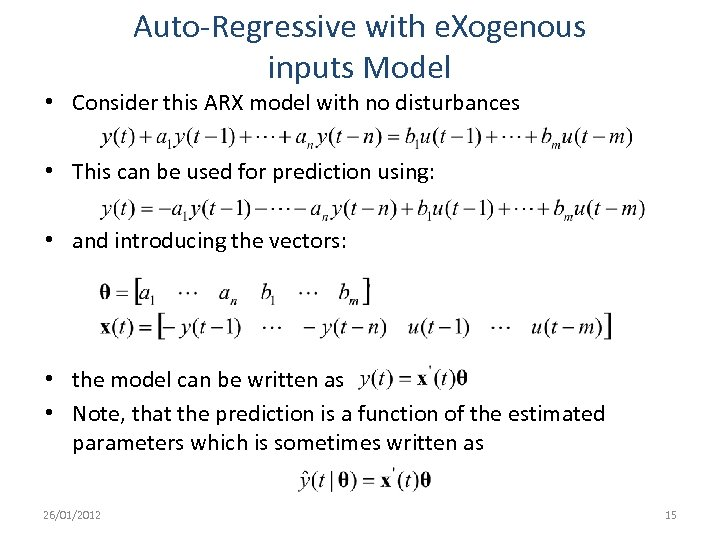Auto-Regressive with e. Xogenous inputs Model • Consider this ARX model with no disturbances • This can be used for prediction using: • and introducing the vectors: • the model can be written as • Note, that the prediction is a function of the estimated parameters which is sometimes written as 26/01/2012 15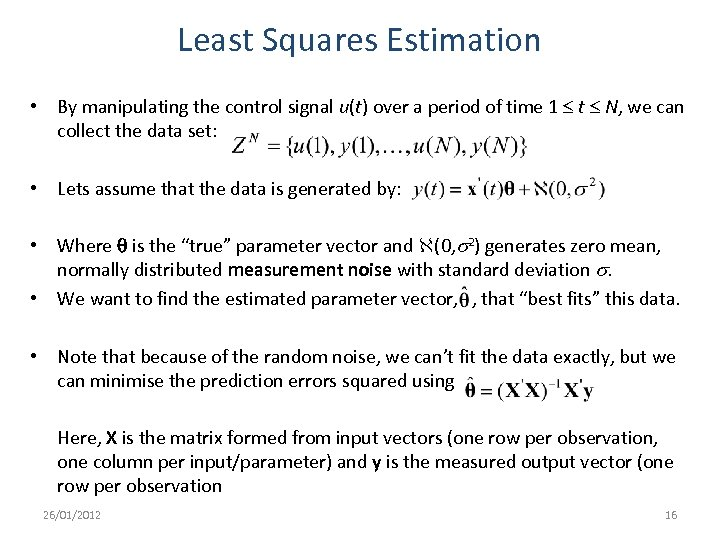Least Squares Estimation • By manipulating the control signal u(t) over a period of time 1 t N, we can collect the data set: • Lets assume that the data is generated by: • Where q is the “true” parameter vector and (0, s 2) generates zero mean, normally distributed measurement noise with standard deviation s. • We want to find the estimated parameter vector, , that “best fits” this data. • Note that because of the random noise, we can’t fit the data exactly, but we can minimise the prediction errors squared using Here, X is the matrix formed from input vectors (one row per observation, one column per input/parameter) and y is the measured output vector (one row per observation 26/01/2012 16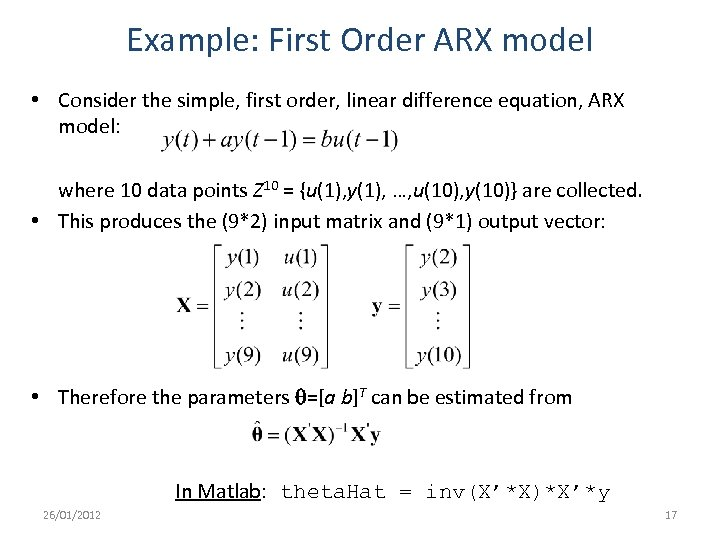Example: First Order ARX model • Consider the simple, first order, linear difference equation, ARX model: where 10 data points Z 10 = {u(1), y(1), …, u(10), y(10)} are collected. • This produces the (9*2) input matrix and (9*1) output vector: • Therefore the parameters q=[a b]T can be estimated from In Matlab: theta. Hat = inv(X’*X)*X’*y 26/01/2012 17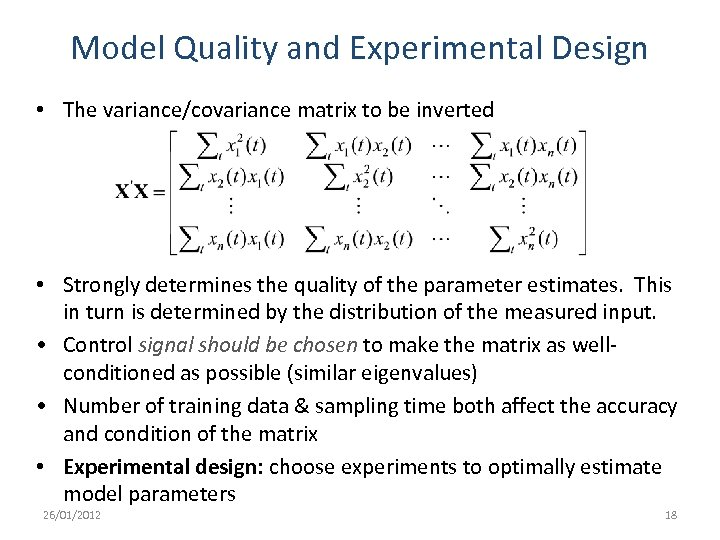Model Quality and Experimental Design • The variance/covariance matrix to be inverted • Strongly determines the quality of the parameter estimates. This in turn is determined by the distribution of the measured input. • Control signal should be chosen to make the matrix as wellconditioned as possible (similar eigenvalues) • Number of training data & sampling time both affect the accuracy and condition of the matrix • Experimental design: choose experiments to optimally estimate model parameters 26/01/2012 18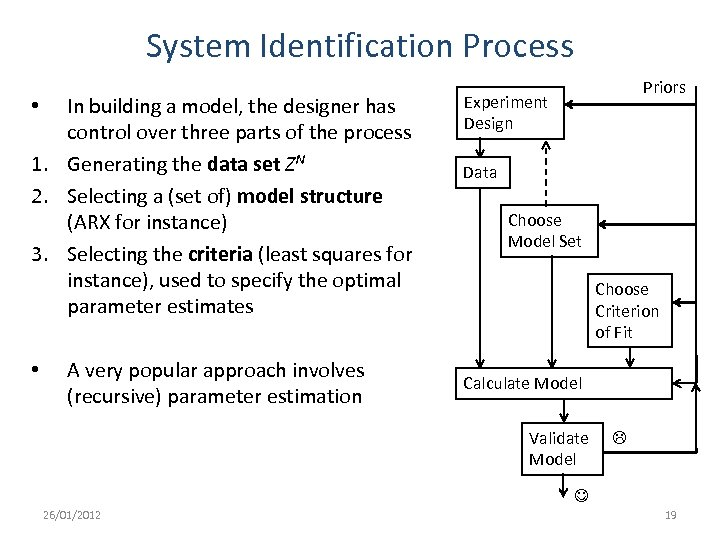System Identification Process In building a model, the designer has control over three parts of the process 1. Generating the data set ZN 2. Selecting a (set of) model structure (ARX for instance) 3. Selecting the criteria (least squares for instance), used to specify the optimal parameter estimates • • A very popular approach involves (recursive) parameter estimation Priors Experiment Design Data Choose Model Set Choose Criterion of Fit Calculate Model Validate Model 26/01/2012 19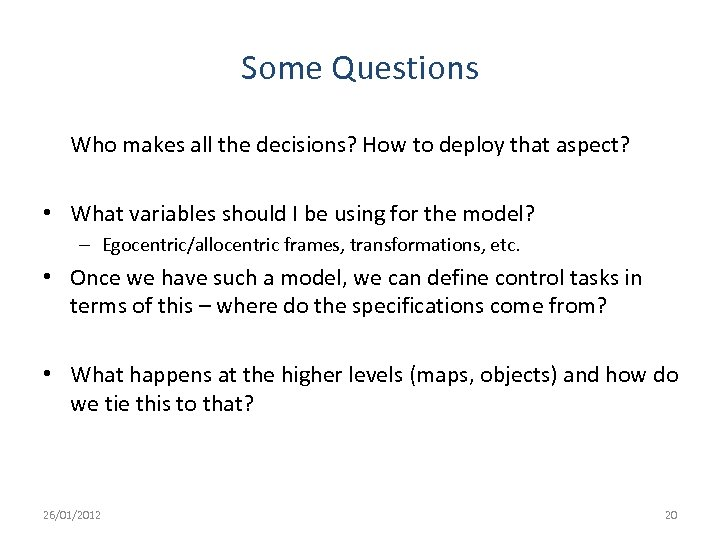Some Questions Who makes all the decisions? How to deploy that aspect? • What variables should I be using for the model? – Egocentric/allocentric frames, transformations, etc. • Once we have such a model, we can define control tasks in terms of this – where do the specifications come from? • What happens at the higher levels (maps, objects) and how do we tie this to that? 26/01/2012 20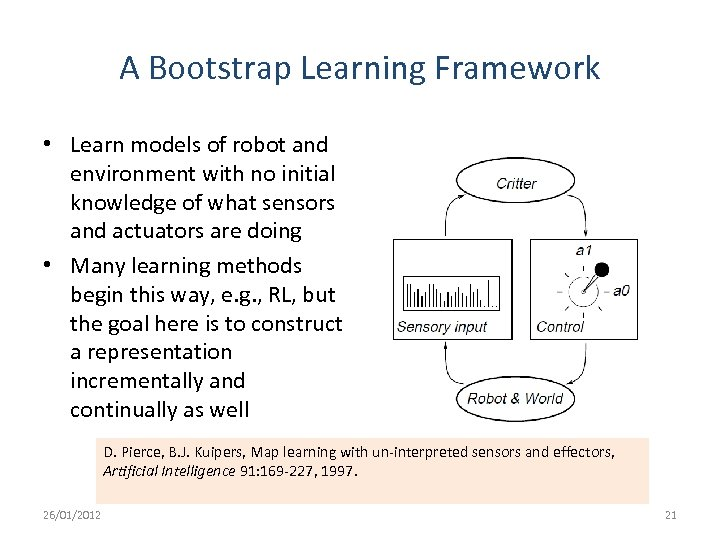A Bootstrap Learning Framework • Learn models of robot and environment with no initial knowledge of what sensors and actuators are doing • Many learning methods begin this way, e. g. , RL, but the goal here is to construct a representation incrementally and continually as well D. Pierce, B. J. Kuipers, Map learning with un-interpreted sensors and effectors, Artificial Intelligence 91: 169 -227, 1997. 26/01/2012 21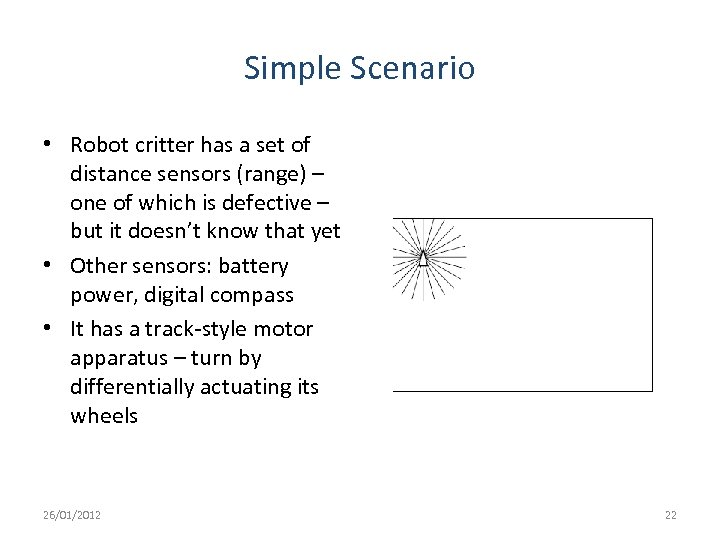Simple Scenario • Robot critter has a set of distance sensors (range) – one of which is defective – but it doesn’t know that yet • Other sensors: battery power, digital compass • It has a track-style motor apparatus – turn by differentially actuating its wheels 26/01/2012 22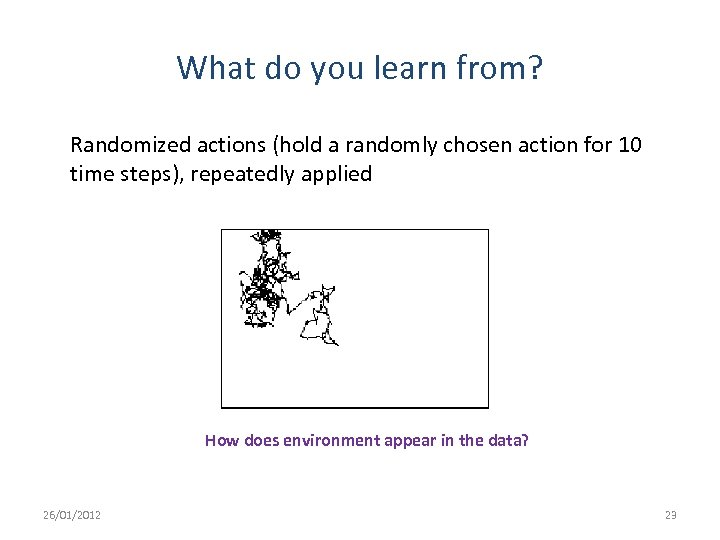What do you learn from? Randomized actions (hold a randomly chosen action for 10 time steps), repeatedly applied How does environment appear in the data? 26/01/2012 23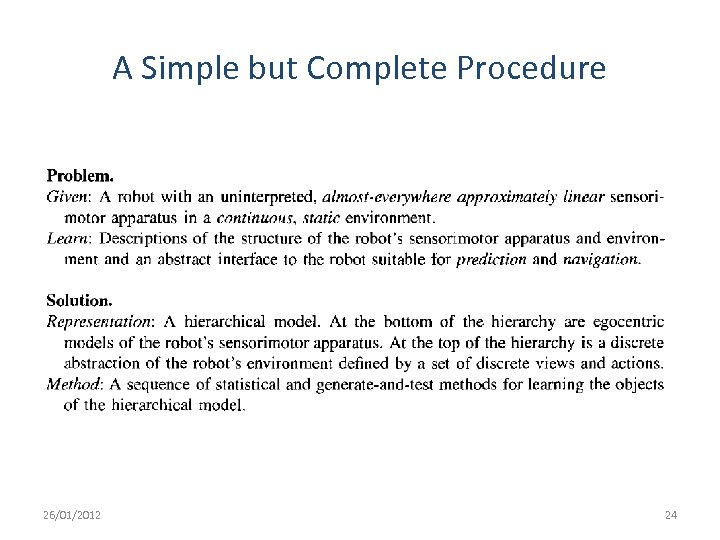A Simple but Complete Procedure 26/01/2012 24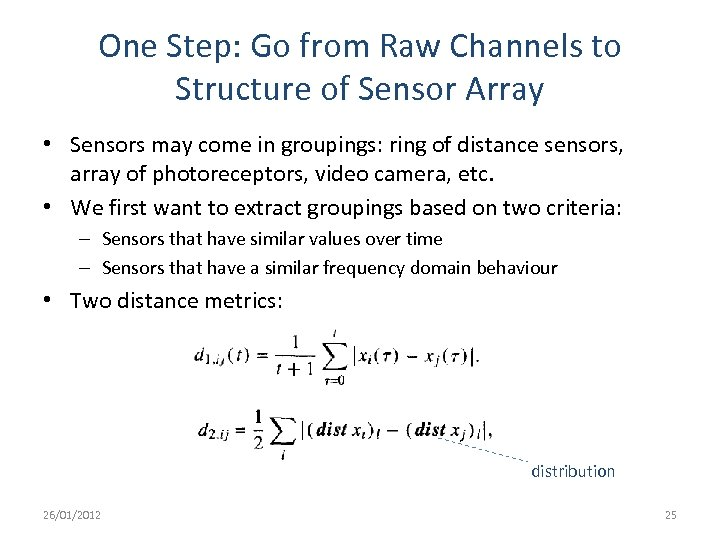One Step: Go from Raw Channels to Structure of Sensor Array • Sensors may come in groupings: ring of distance sensors, array of photoreceptors, video camera, etc. • We first want to extract groupings based on two criteria: – Sensors that have similar values over time – Sensors that have a similar frequency domain behaviour • Two distance metrics: distribution 26/01/2012 25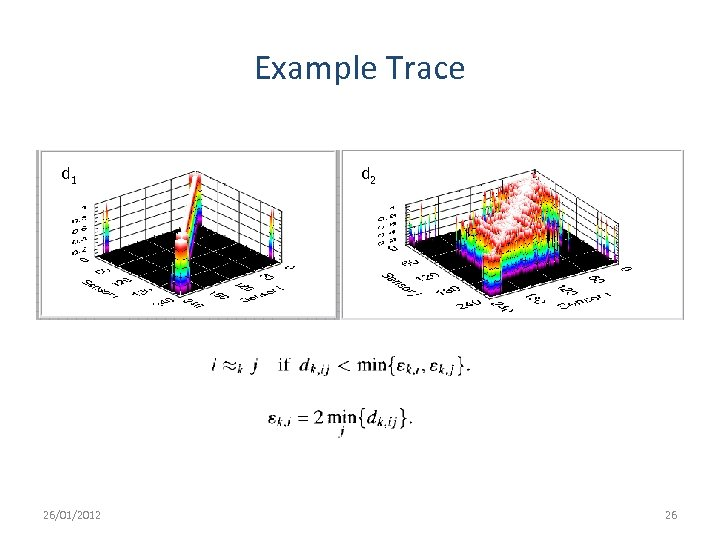Example Trace d 1 26/01/2012 d 2 26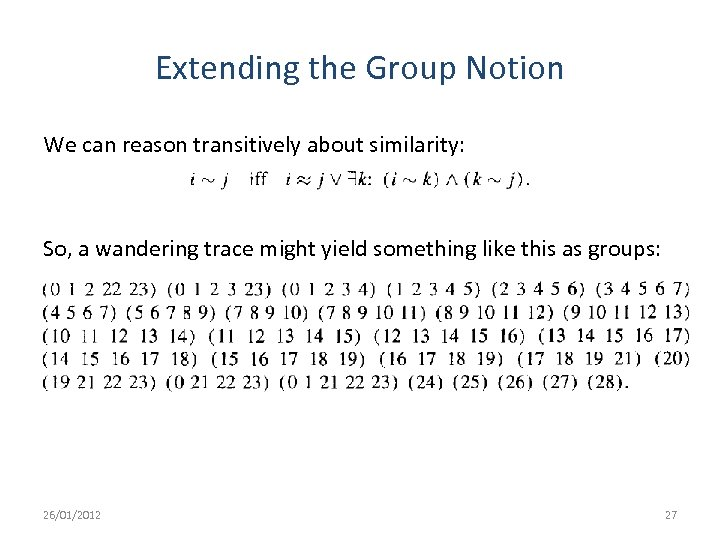Extending the Group Notion We can reason transitively about similarity: So, a wandering trace might yield something like this as groups: 26/01/2012 27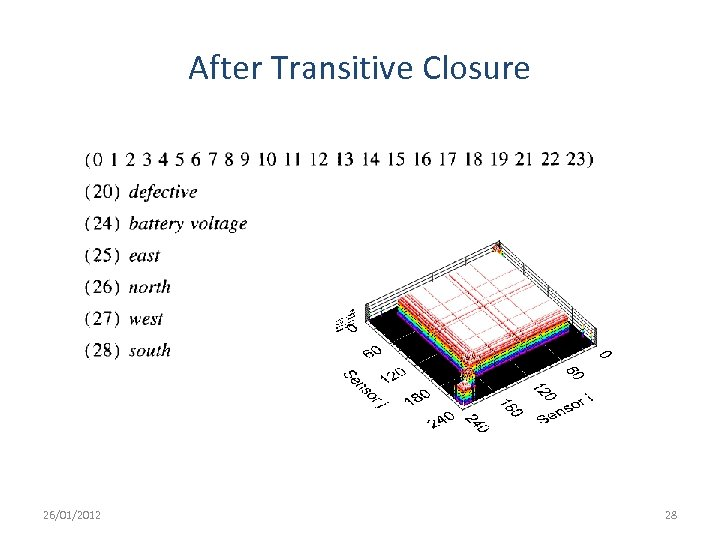After Transitive Closure 26/01/2012 28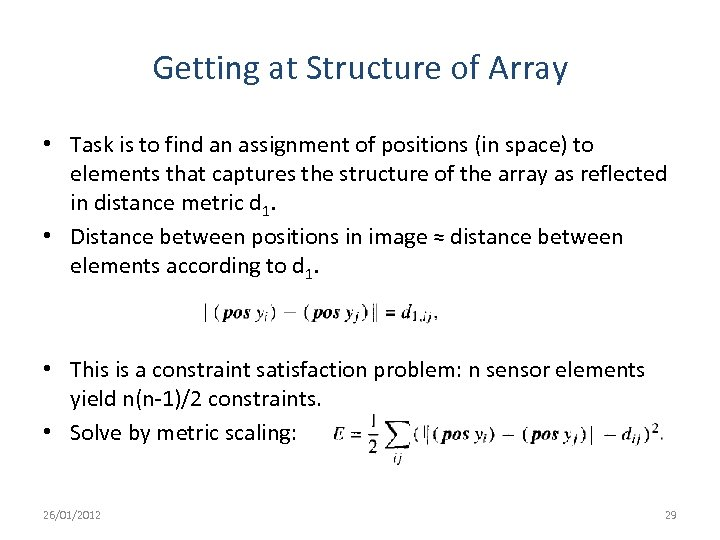Getting at Structure of Array • Task is to find an assignment of positions (in space) to elements that captures the structure of the array as reflected in distance metric d 1. • Distance between positions in image ≈ distance between elements according to d 1. • This is a constraint satisfaction problem: n sensor elements yield n(n-1)/2 constraints. • Solve by metric scaling: 26/01/2012 29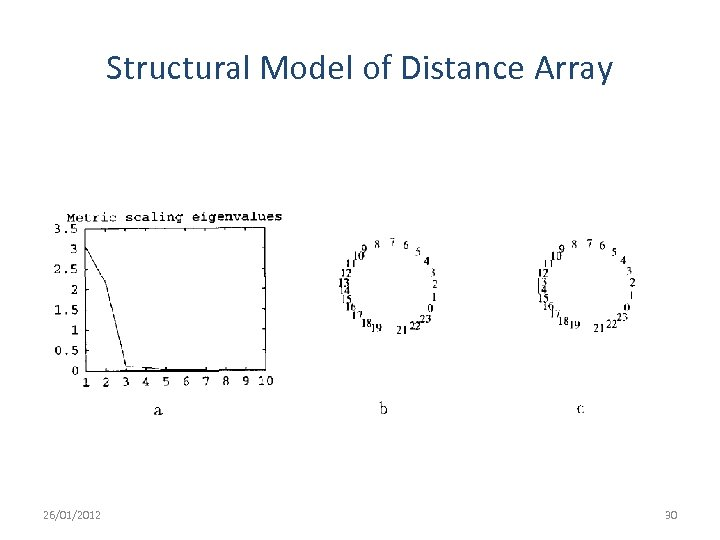Structural Model of Distance Array 26/01/2012 30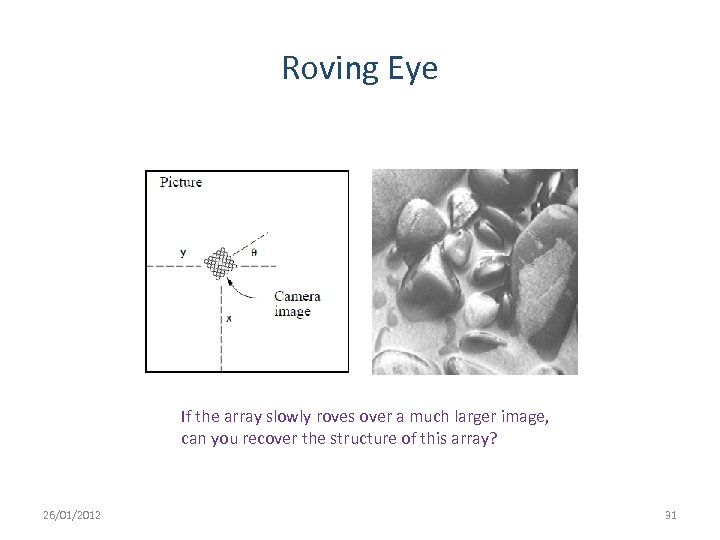Roving Eye If the array slowly roves over a much larger image, can you recover the structure of this array? 26/01/2012 31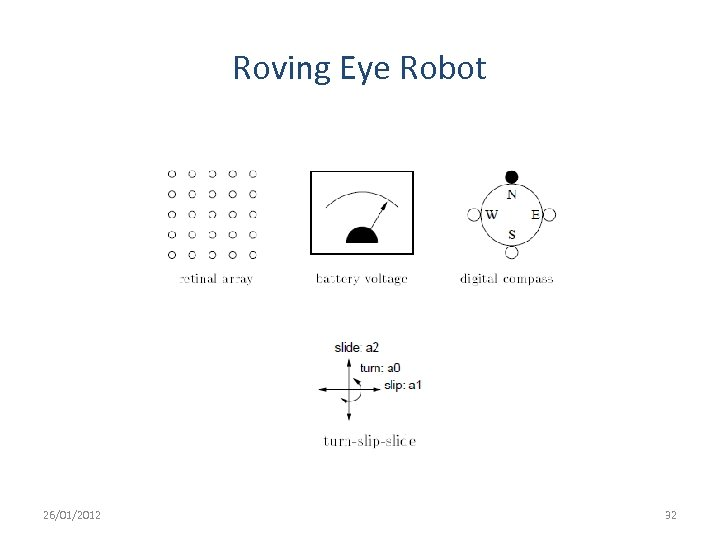Roving Eye Robot 26/01/2012 32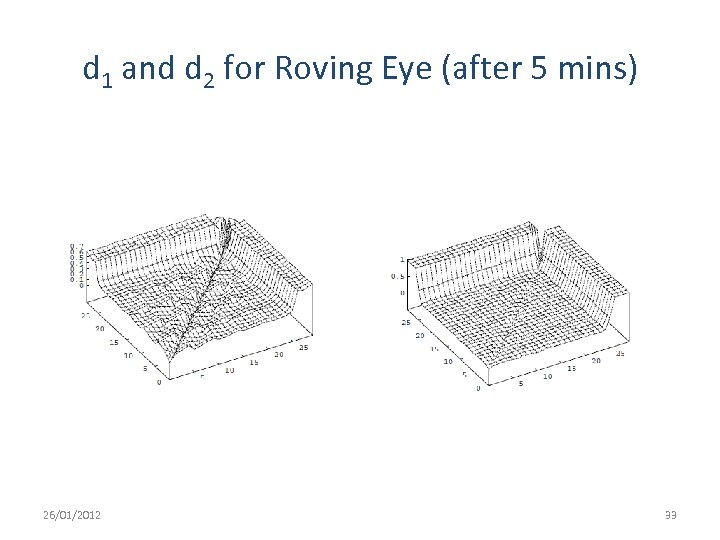d 1 and d 2 for Roving Eye (after 5 mins) 26/01/2012 33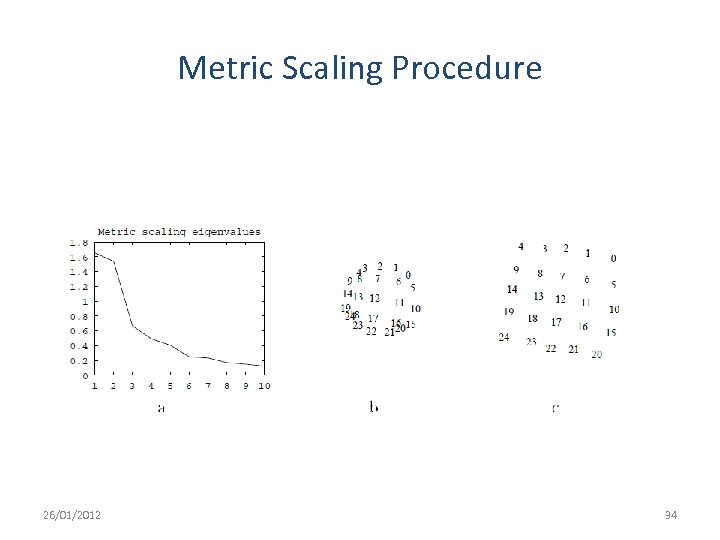Metric Scaling Procedure 26/01/2012 34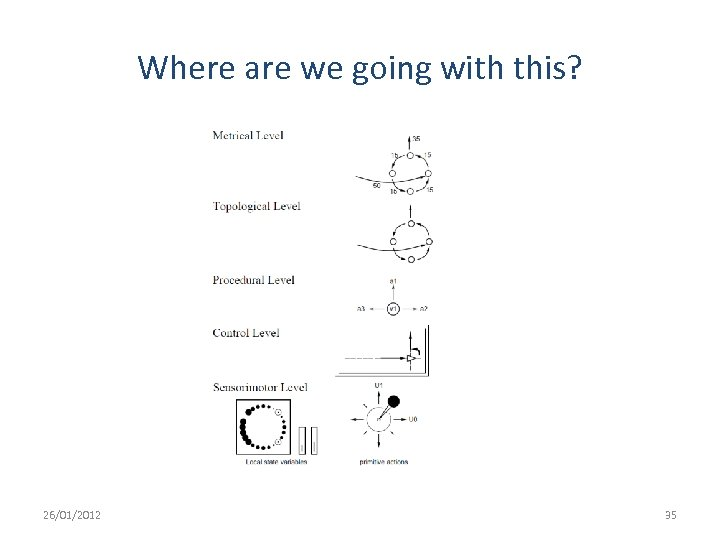Where are we going with this? 26/01/2012 35